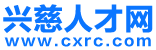• 微信
• 登录 | 注册• 不限
• 普工技工
• 生产研发
• 文职文员
• 行政人事
• 会计财务
• 物流贸易
• 质控安防
• 电子电气
• 市场销售
• 建筑装修
• 酒店餐饮
• 百货零售
• 客户服务
• 家政保洁
• 保安司机
• 淘宝电商
• 汽车服务
• 教育培训
• 高级管理
• 娱乐休闲
• 医疗保健
• 旅游健身
• 物业管理
• 编辑印刷
• 设计包装
• 机械仪表
• 轻工工艺
• 网络通信
• 化工制药
• 广告会展
• 能源环保
• 美容美发
• 摄影影视
• 房产中介
• 咨询顾问
• 翻译法律拍卖
• 金融保险
• 农林牧渔
• 其他分类

• 不限
• 财务主管/经理
• 会计
• 财务/会计助理
• 注册会计师
• 注册审计师
• 审计
• 总账主任
• 出纳
• 审计(核)主管/经理
• 统计
• 财务分析
• 成本分析/核算
• 帐目(进出口)管理
• 营运会计经理
• 审计会计经理
• 财务总监
• 税务专员/助理
• 税务经理/主管
• 财务分析员
• 成本管理员
• 会计财务其他相关职位

• 慈溪市
• 不限
• 浒山街道
• 白沙路街道
• 宗汉街道
• 坎墩街道
• 古塘街道
• 杭州湾新区
• 庵东镇
• 横河镇
• 匡堰镇
• 崇寿镇
• 观海卫镇
• 龙山镇
• 掌起镇
• 周巷镇
• 长河镇
• 逍林镇
• 胜山镇
• 新浦镇
• 附海镇
• 桥头镇
• 余姚市
• 外地

• 不限
• 今天
• 三天内
• 一周内
• 一个月内
• 三个月内

• 不限
• 全职
• 兼职

• 不限
• 1000元以下
• 1000-2000元
• 2000-3000元
• 3000-5000元
• 5000-8000元
• 8000-1.2万元
• 1.2万-2万元
• 2万元以上
• 面议